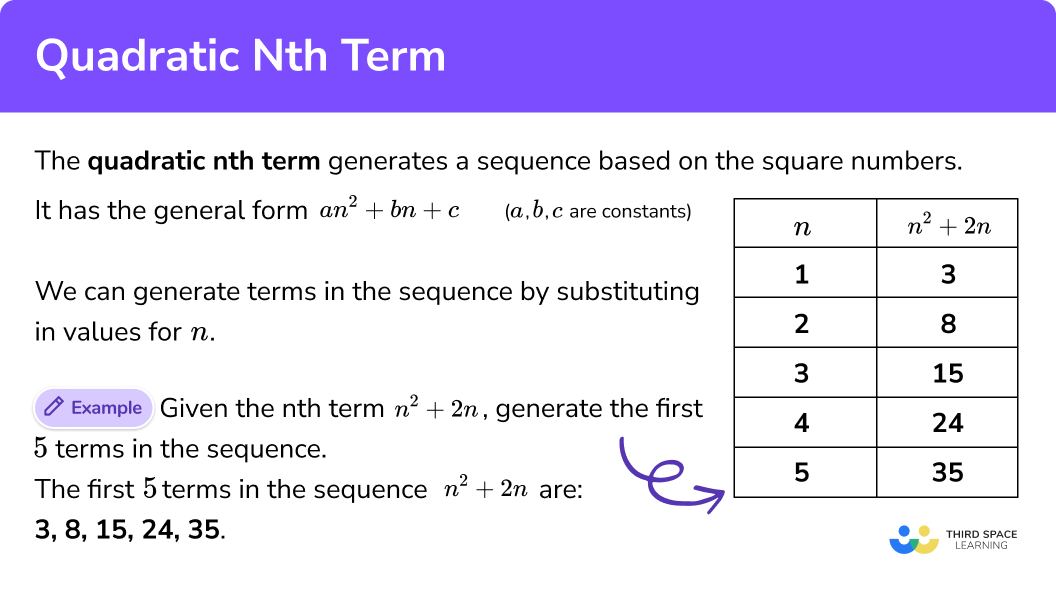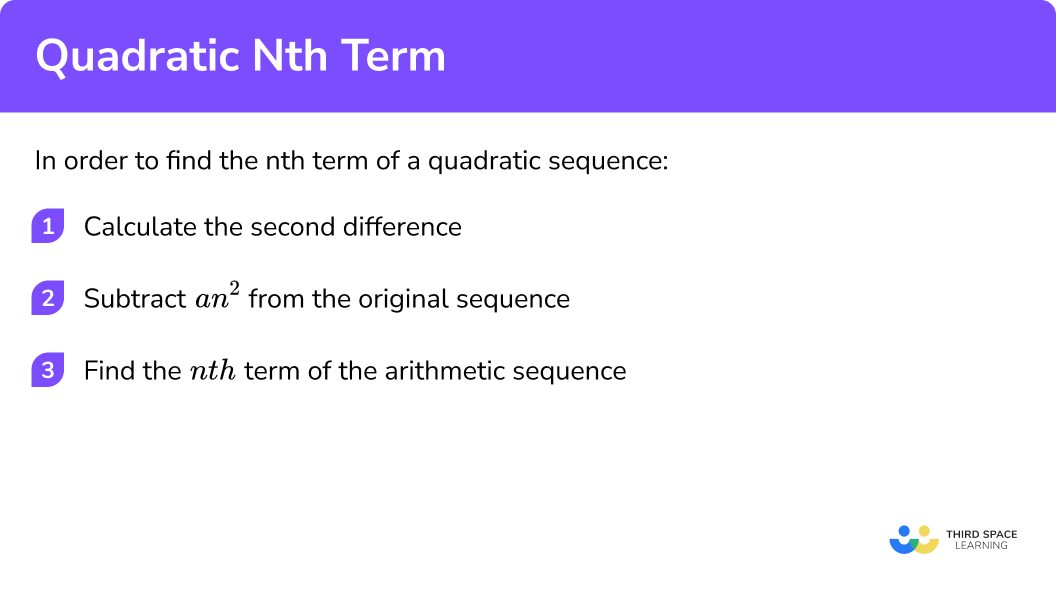Here we will learn about the nth term of a quadratic sequence, including generating a quadratic sequence, finding the nth term of a quadratic sequence and applying this to real life problems.

There are also quadratic nth term worksheets based on Edexcel, AQA and OCR exam questions, along with further guidance on where to go next if you’re still stuck.

## What is a quadratic nth term?

A quadratic nth term is a rule used to generate a sequence based on the square numbers and has the general form an^{2}+bn+c where a, b, and c are constants (a constant is a number that does not change).

This looks very similar to a quadratic expression (ax^{2}+bx+c) however by substituting in values for n we generate a sequence where the common difference between two terms is not the same for any two terms.

E.g.

Given the n^{th} term n^{2}+2n , generate the first 5 terms in the sequence.

So the first 5 terms in the sequence with the nth term rule n^{2}+2n are: 3, 8, 15, 24, 35.

Let us inspect the sequence of n^{2} .

If we write the first 5 terms of the sequence of n^{2}, we get the numbers: 1, 4, 9, 16, 25 . This is because when you substitute the values of 1, 2, 3, 4, and 5 into the nth term, we get the first 5 square numbers.

Let’s look at the term to term difference.

Here, the first term to term difference is +3, +5, +7, +9 , etc and so the sequence n^{2} increases by another sequence. We can therefore use this sequence as a framework when trying to find the nth term of a quadratic sequence.

What if we had the sequence 2n^{2} ?

The first 5 terms of the sequence 2n^{2} are 2, 8, 18, 32, 50 . This is calculated by multiplying the terms for the sequence n^{2} by 2 .

Notice that the term to term difference is still not the same:

The term to term differences for 2n^{2} are now twice as large as the term to term difference for n^{2} .

This means that when you see a sequence that has a difference based on the numbers +3, +5, +7, +9 , this means that the sequence is based on the square numbers, and so your sequence will be a quadratic sequence of the form an^{2}+bn+c .

Let us now reverse the question previously and use the first 5 terms in the sequence 3, 8, 15, 24, 35 to find the nth term of the sequence.

So we have the sequence: 3, 8, 15, 24, 35 .

If we look at the term to term difference between these numbers, we have:

Notice that there is no common difference from one term to the next so this sequence is based on the sequence n^{2} .

We have a small problem though as even though this sequence increases by +5, +7, +9, +11, etc, we do not know what the coefficient of n^{2} is (the value of a for an^{2} ).

To work this out, we need to halve the second difference.

The second difference is the term to term rule between the first difference. For our sequence above we have:

The second difference for this sequence is +2 , and so as the coefficient of n^{2} is half of this number, we can now state that our sequence is based on the sequence 1n^{2} or just n^{2}=1, 4, 9, 16, 25.

So how do we get from the numbers: 1, 4, 9, 16, 25 to the terms in the sequence 3, 8, 14, 24, 35 ?

We start by finding the remainder between each term:

So now we have a new sequence of numbers: 2, 4, 6, 8, 10.

We can use our knowledge of finding the nth term of an arithmetic sequence to work out the nth term to be 2n .

So nth term of our quadratic sequence is n^{2}+2n .

### What is a quadratic nth term?## How to find the nth term of a quadratic sequence

In order to find the nth term of a quadratic sequence:

1. Calculate the second difference.
2. Subtract \bf{an^{2}} from the original sequence.
3. Find the \textbf{nth} term of the arithmetic sequence.

### Explain how to find the nth term of a quadratic sequence### Related lessons on sequences

Quadratic nth term is part of our series of lessons to support revision on sequences. You may find it helpful to start with the main sequences lesson for a summary of what to expect, or use the step by step guides below for further detail on individual topics. Other lessons in this series include:

### Example 1: an2

Calculate the nth term for the following sequence: 4, 16, 36, 64, 100

1. Calculate the second difference.

The second difference is +8 . By halving the second difference, the sequence is based on 4n^{2} .

2Subtract an^{2} from the original sequence.

3Find the nth term of the arithmetic sequence.

As the remainder is 0 for each term, there is no arithmetic sequence to find the nth term of and so the nth term of the sequence 4, 16, 36, 64, 100 is:
4n^{2} .

### Example 2: an2

Calculate the nth term for the following sequence: −5, −20, −45, −80, −125 .

The second difference is −10 . By halving the second difference, the sequence is based on −5n^{2} .

As the remainder is 0 for each term, there is no arithmetic sequence to find the nth term of and so the nth term of the sequence −5 , −20 , −45 , −80 , −125 is:
−5n^{2} .

### Example 3: an2+bn

Calculate the nth term for the following sequence: 7, 20, 39, 64, 95 .

The second difference is +6 . By halving the second difference, the sequence is based on 3n^{2} .

We now have the remaining arithmetic sequence 4, 8, 12, 16, 20.

The nth term of the sequence 4, 8, 12, 16, 20 is 4n , so the nth term of this quadratic sequence 7, 20, 39, 64, 95 is:
3n^{2}+4n.

### Example 4: an2+c

Calculate the nth term for the following sequence: 1, −5, −15, −29, −47

The second difference is −4 . By halving the second difference, the sequence is based on −2n^{2} .

The remainder is a constant and so there is no arithmetic sequence to calculate the nth term of. We just add 3 to the value of −2n^{2} .

The nth term of the sequence 1, -5, -15, -29, -47 is:
-2n^{2}+3 .

### Example 5: an2+bn+c

Calculate the nth term for the following sequence: 7, 14, 23, 34, 47

The second difference is +2 . By halving the second difference, the sequence is based on n^{2} .

The remainder is an arithmetic sequence 6, 10, 14, 18, 22.

The nth term of the arithmetic sequence is 4n+2 . This means that the nth term of our quadratic sequence is:
n^{2}+4n+2 .

### Example 6: an2+bn+c

Calculate the nth term for the following sequence: −5, −1, 9, 25, 47

The second difference is +3 . By halving the second difference, the sequence is based on 3n^{2} .

The remainder is an arithmetic sequence −8, −13, −18, −23, −28

The nth term of the arithmetic sequence −8, −13, −18, −23, −28 is −5n−3 so the nth term of our quadratic sequence is:
3n^{2}−5n−3 .

### Common misconceptions

• Second difference

A common error is to forget to half the second difference before using it as the coefficient of n^{2} .
E.g.
For the quadratic sequence n^{2}=1, 4, 9, 16, 25 , …the second difference is equal to 2 and so the nth term could incorrectly written as 2n^{2} .

• Confusing a quadratic sequence with an arithmetic sequence

A quadratic sequence will have a common second difference.

• Positive and negative second differences

When the sequence is decreasing into negative terms, the second difference can be written incorrectly as a positive number.
E.g.
The sequence −n^{2} = −1, −4, −9, −16, … has a second difference of −2 but is incorrectly written as 2 .

• Values of n

The value for n cannot be negative. n represents the term (position) numbers and therefore it can only be positive integers starting from 1 and should also not include 0, n=1, 2, 3, 4, 5, ….

This is important when finding the term in the sequence given its value as a zero or negative solution for n can be calculated.

### Practice quadratic nth term questions

1. Calculate the nth term of the quadratic sequence: 2,8,18,32,50

6n−46n^24n^22n^2Second difference +4
2n^2=2, 8, 18, 32, 50

2. Calculate the nth term of the quadratic sequence: −3, −12, −27, −48, −75

−3n(−3n)^2−3n^2−4.5n^2Second difference −6
−3n^2= −3, −12, −27, −48, −75

3. Calculate the nth term of the quadratic sequence: 7, 24, 51, 88, 135

5n+25n^2+2n5n^2−2n10n^2−3Second difference +10

 Original 7 24 51 88 135 5n2 5 20 45 80 125 Original – 5n2 2 4 6 8 10
2n=2,4,6,8,10

So nth term = 5n^2+2n

4. Calculate the nth term of the quadratic sequence: −10, −22, −42, −70, −106

−4n−6−4n^2+6−8n^2−2−4n^2−6Second difference −8
−4n^2= −4, −16, −36, −64, −100

 Original -10 -22 -42 -70 -106 4n2 -4 -16 -36 -64 -100 Original – 4n2 -6 -6 -6 -6 -6

So nth term = 4n^2 – 6

5. Calculate the nth term of the quadratic sequence: 3, 11, 23, 39, 59

2n+12n^2+12n^2+2n-14n^2-1Second difference +4
2n^2 = 2, 8, 18, 32, 50

 Original 3 11 23 39 59 2n2 2 8 18 32 50 Original – 2n2 1 3 5 7 9
2n – 1 =1, 3, 5, 7, 9

So nth term = 2n^2 + 2n – 1

6. Calculate the nth term of the quadratic sequence: 6, 15, 32, 57, 90

4n+24n^2-3n+54n^2+3n-58n^2-2Second difference +8
4n^2=4, 16, 36, 64, 100

 Original 6 15 32 57 90 4n2 4 16 36 64 100 Original – 4n2 2 -1 -4 -7 -10
-3n + 5 = 2, -1, -4, -7, -10

So nth term = 4n^2 – 3n + 5

### Quadratic nth term GCSE questions

1. Given that the nth term of the sequence 2, 8, 18, 32, 50 is 2n^2 , state the nth term of the sequence 3, 10, 21, 36, 55 .

(2 marks)

Remainder: 1, 2, 3, 4, 5

(1)

nth term: 2n^2+n

(1)

2. (a) Given the first three terms in the sequence are 2, 18, 48 , state the next two terms in the sequence.
(b) Calculate the nth term of the sequence

(5 marks)

92

(1)

150

(1)

7n^2=7, 28, 63, 112, 175

(1)

5n=5,10,15,20,25

(1)

nth term = 7n^2-5n

(1)

3. Is the value 52 a term in the sequence n^2+3n+2 ?

(4 marks)

n^2+3n+2=52 so n^2+3n-50=0

(1)

Factor pairs of 50 : 1×50, 2×25, 5×10

(1)

Does not factorise

(1)

No

(1)

## Learning checklist

You have now learned how to:

• Deduce expressions to calculate the nth term of quadratic sequences

## Still stuck?

Prepare your KS4 students for maths GCSEs success with Third Space Learning. Weekly online one to one GCSE maths revision lessons delivered by expert maths tutors.

Find out more about our GCSE maths tuition programme.